# Position of particles on a detector after decay

• Cocoleia
In summary, the code to generate an angular distribution for p- and 3He decay products will need to assume an angular distribution in the c.o.m. system and then transfom to the detector system.

## Homework Statement

A hypertriton (a bound system with a L hyperon together with a deuteron core (proton
and neutron) is produced at the origin of the coordinate, (x,y)=(0,0) with a velocity of 0.94c
(beta=0.94), flying along the x-axis. The mass of the hypertriton is 2.991 GeV/c2

. It decays into pi (mp- = 0.14 GeV/c2) and 3He (m3He = 2.809 GeV/c2). The hypertriton immediately decays at the origin. Now you have a detector at x=0.4. Create y-hit distributions of p- and 3He with
10000 hypertriton decay events.

## The Attempt at a Solution

I am writing a code to do this, but I am having trouble understanding.
I will be randomly generating angles (only forwards) for my particles in this code.
I am thinking, if I know the angle of the particle and the distance in x that it travels (0.4m) then can I just use a simple sin (basic geometry) to find the position in why
so y = sin(theta) * (0.4)

I feel like this is way too simple so I am missing something crucial in the question

Cocoleia said:
I will be randomly generating angles (only forwards) for my particles in this code.
In the center of mass system of the hyperthing, the decay products will fly off in back to back directions, so you can't just 'generate random angles' for your particles. You have to assume an angular distribution in the c.o.m. system and then transfom to the detector system.

BvU said:
In the center of mass system of the hyperthing, the decay products will fly off in back to back directions, so you can't just 'generate random angles' for your particles. You have to assume an angular distribution in the c.o.m. system and then transfom to the detector system.
Okay, and once I do this, I will have angles that I will be able to use? Is my logic for just doing sin(theta)*x correct? Or there will be some more lorentz transformation type things involved ?

Yes, transform means Lorentz transform. Transverse momentum is only from the decay, longitudinal from c.o.m. motion + decay.

BvU said:
Yes, transform means Lorentz transform. Transverse momentum is only from the decay, longitudinal from c.o.m. motion + decay.
So I will need to use something like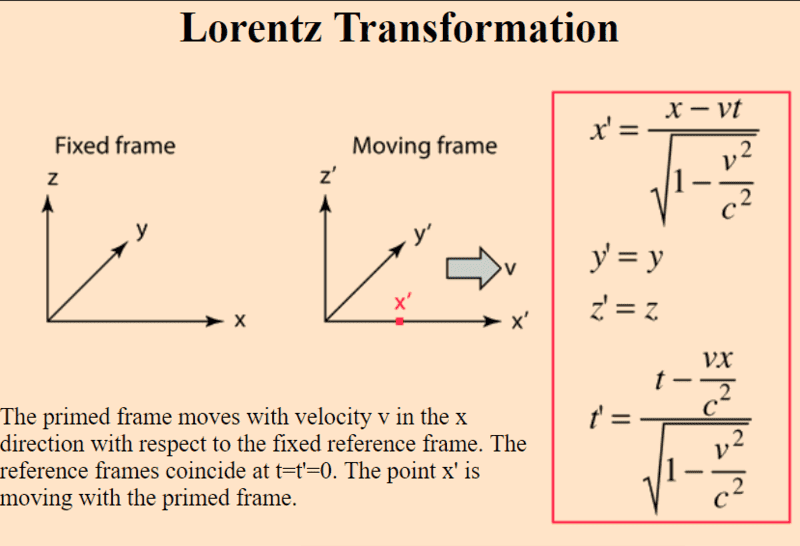But if the decay happens at the origin, then it is not traveling in the moving frame and therefore this would not be applicable?

Let's say in my second example it travels 180 picoseconds before decaying. Then I would find x' by this formula and use that to find the distance with the sin(theta)?

#### Attachments

Last edited:
http://www.helsinki.fi/~www_sefo/phenomenology/Schlippe_relativistic_kinematics.pdf

BvU said:
http://www.helsinki.fi/~www_sefo/phenomenology/Schlippe_relativistic_kinematics.pdf
I have been reading a few things, let's say https://hepweb.ucsd.edu/ph110b/110b_notes/node43.html
So if the lifetime is 0, then it doesn't make much of a difference for the reference frames right ?
I have already derived all equations for momentum and energy, I'm confused how to consider the position

BvU said:
http://www.helsinki.fi/~www_sefo/phenomenology/Schlippe_relativistic_kinematics.pdf
Let's say I take my second example, where the hypertriton lives for 180 picoseconds before decaying into the pion and helium. Obviously, it will not decay at the origin anymore since it travels somewhere during that lifetime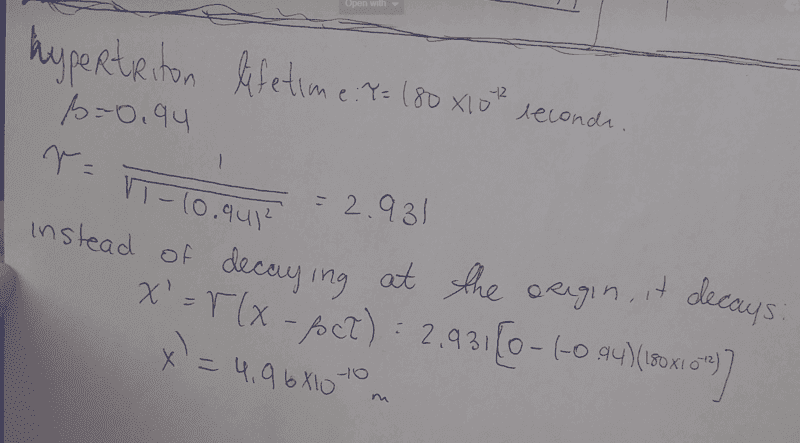This is what I did. Does it make sense? Now I know it decays at x=4.96x10^-10 and can use that for my calculations (with the angles, etc)
So it would travel 0.3999999995m in the x direction, at whatever angle theta (suppose it is known) AND i could do tan(theta) * x = y to find which point it hits on the detector

#### Attachments

Last edited:
Cocoleia said:
So if the lifetime is 0, then it doesn't make much of a difference for the reference frames right ?
What do you mean: "if" ? The problem statement says so (to make it easier for you)
Cocoleia said:
The hypertriton immediately decays at the origin

Cocoleia said:
I have already derived all equations for momentum and energy, I'm confused how to consider the position
You don't need the position. You need angles.

Re lifetime: I get ##\gamma = ## 2.9 too. So in the lab it lives 2.9 * 180 ps ##\approx## 0.5 ns. That is 15 cm. Looks to me as if you mix up frames of reference or something. Aha:

Cocoleia said:
So it would travel 0.3999999995m in the x direction, at whatever angle theta
What's this calculation ? Your calculation to get 5e-10 misses by a factor of ##c## !
How do you get 0.4 m ? (not 0.3999999995 !) Aha: subtract 5e-10 (from 0.4 ##\pm ## ??) -- I was under the impression 'it' is the hyperthing, not the decay products.

(and your ##\gamma## looks uncomfortably similar to a ##\sqrt{}##)

So not 0.5 nm but 15 cm (with a Poisson distribution! It is a decay process!), and in the x direction (before decay it does not deflect).

However, to make it easier for you they set the lifetime to 0 and thereby the decay at (0,0). At least that's how I read the problem statement, but you may have better information... (is this really an exercise, intro physics homework ? )

Now generate an angular distribution for the momenta in the com and transform them to the lab.

Study the link in #6; it's a lot better than the ucsd oneBvU said:
What do you mean: "if" ? The problem statement says so (to make it easier for you)

You don't need the position. You need angles.

Re lifetime: I get ##\gamma = ## 2.9 too. So in the lab it lives 2.9 * 180 ps ##\approx## 0.5 ns. That is 15 cm. Looks to me as if you mix up frames of reference or something. Aha:

What's this calculation ? Your calculation to get 5e-10 misses by a factor of ##c## !
How do you get 0.4 m ? (not 0.3999999995 !) Aha: subtract 5e-10 (from 0.4 ##\pm ## ??) -- I was under the impression 'it' is the hyperthing, not the decay products.

(and your ##\gamma## looks uncomfortably similar to a ##\sqrt{}##)

So not 0.5 nm but 15 cm (with a Poisson distribution! It is a decay process!), and in the x direction (before decay it does not deflect).

However, to make it easier for you they set the lifetime to 0 and thereby the decay at (0,0). At least that's how I read the problem statement, but you may have better information... (is this really an exercise, intro physics homework ? )

Now generate an angular distribution for the momenta in the com and transform them to the lab.

Study the link in #6; it's a lot better than the ucsd oneYes, I have everything mixed up.

I have one first problem where the lifetime is 0 and another where it is 180. I was just comparing them to see if I understood the general concept.

I mean, I am at the level of introduction to physics but that's potentially not the work I'm given. It's not homework though, it won't be graded. It's actual workI will work on this and read the other document more thoroughly and check backBvU said:
Now generate an angular distribution for the momenta in the com and transform them to the lab.

Study the link in #6; it's a lot better than the ucsd oneJust out of curiosity, once I have the lab frame momentum how do I convert this to the position on the detector (how do i find where they will hit)

BvU said:
Now generate an angular distribution for the momenta in the com and transform them to the lab.

Study the link in #6; it's a lot better than the ucsd oneIs this what you mean by angular distribution of momentum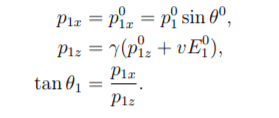with (0) being in the rest frame

Cocoleia said:

## Homework Statement

A hypertriton (a bound system with a L hyperon together with a deuteron core (proton
and neutron) is produced at the origin of the coordinate, (x,y)=(0,0) with a velocity of 0.94c
(beta=0.94), flying along the x-axis. The mass of the hypertriton is 2.991 GeV/c2

. It decays into pi (mp- = 0.14 GeV/c2) and 3He (m3He = 2.809 GeV/c2). The hypertriton immediately decays at the origin. Now you have a detector at x=0.4. Create y-hit distributions of p- and 3He with
10000 hypertriton decay events.

## The Attempt at a Solution

I am writing a code to do this, but I am having trouble understanding.
I will be randomly generating angles (only forwards) for my particles in this code.
I am thinking, if I know the angle of the particle and the distance in x that it travels (0.4m) then can I just use a simple sin (basic geometry) to find the position in why
so y = sin(theta) * (0.4)

I feel like this is way too simple so I am missing something crucial in the question

When you say you are "writing a code", what do you mean? The problem looks like a fairly standard one involving the calculation of a probability density function under a transformation (that is, a change of variables). A closed-form analytical solution looks perfectly feasible, but only if you have taken some material about probability densities and the like. If that material is totally new to you I could see the virtue of something like a Monte-Carlo simulation, to obtain an "experimental" y-distribution.

Last edited:
Ray Vickson said:
When you say you are "writing a code", what do you mean? The problem looks like a fairly standard one involving the calculation of a probability density function under a transformation (that is, a change of variables). A closed-form analytical solution looks perfectly feasible, but only if you have taken some material about probability densities and the like. If that material is totally new to you I could see the virtue of something like a Monte-Carlo simulation, to obtain an "experimental" y-distribution.
Yes, it's just a first exercise to introduce me to the material and the software. eventually it will be a monte carlo simulation... right now I'm just trying to understand the problem and do some basic C++ coding.

Ray Vickson said:
When you say you are "writing a code", what do you mean? The problem looks like a fairly standard one involving the calculation of a probability density function under a transformation (that is, a change of variables). A closed-form analytical solution looks perfectly feasible, but only if you have taken some material about probability densities and the like. If that material is totally new to you I could see the virtue of something like a Monte-Carlo simulation, to obtain an "experimental" y-distribution.
The thing is I'm very new to this and I don't understand what relationship i am supposed to find between the angle, momentum and the position where my particle will hit on the detector. Will it involve angular momentum ?

No. Stick to the simple kinematics of transforming linear momentum.
I sympathize with the newcomer stress - brings back memories. There's so much everybody - except you - seems to know but is unable to explain in a helpful manner.

BvU said:
No. Stick to the simple kinematics of transforming linear momentum.
I sympathize with the newcomer stress - brings back memories. There's so much everybody - except you - seems to know but is unable to explain in a helpful manner.
Yes I see this...
So I have derived / found the equations for momentum of two body decay: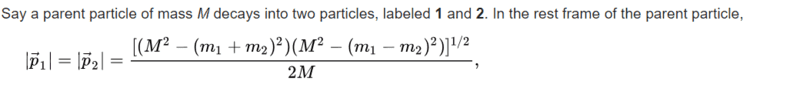Then in the lab frameNow, I also see that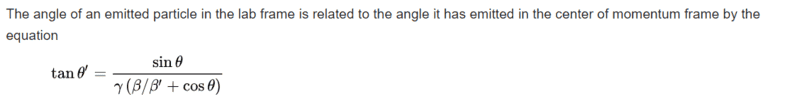I just don't now how to piece this together to get the height at which the particle hits the detector. Somehow use this last formula, tan(theta') * 0.4 will give me the answer I am looking for ?

#### Attachments

BvU said:
No. Stick to the simple kinematics of transforming linear momentum.
I sympathize with the newcomer stress - brings back memories. There's so much everybody - except you - seems to know but is unable to explain in a helpful manner.
Based on this principle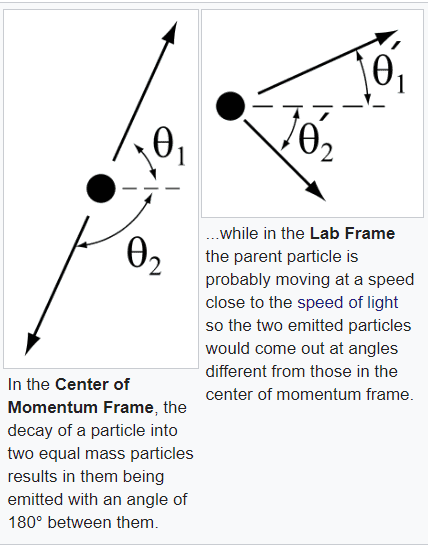#### Attachments

Looks good to me !
(note the striking similarity with Exercise 1 in the helsinki link).

Must say: a little googling nowadays brings to the rescue a huge load of resources !(in the early days of bitnet and earn we would go to the library or try to make a senior colleague explain things a little -- something I would still advise now: very effective and efficient, especially if you butter them up a bit).

BvU said:
Looks good to me !
(note the striking similarity with Exercise 1 in the helsinki link).

Must say: a little googling nowadays brings to the rescue a huge load of resources !(in the early days of bitnet and earn we would go to the library or try to make a senior colleague explain things a little -- something I would still advise now: very effective and efficient, especially if you butter them up a bit).
Thanks for your help. I would love to ask my colleagues for help but everyone is gone this ... just me and my physics. So I turn to Physics ForumsBvU said:
What do you mean: "if" ? The problem statement says so (to make it easier for you)

You don't need the position. You need angles.

Re lifetime: I get ##\gamma = ## 2.9 too. So in the lab it lives 2.9 * 180 ps ##\approx## 0.5 ns. That is 15 cm. Looks to me as if you mix up frames of reference or something. Aha:
Wait, how did you find 15cm ?

Cocoleia said:
The thing is I'm very new to this and I don't understand what relationship i am supposed to find between the angle, momentum and the position where my particle will hit on the detector. Will it involve angular momentum ?

Look at your own diagram in post #18. For any angle ##\theta_1## in the CM frame, particle 1 will either strike the detector or it won't. Clearly, if ##-\pi/2 < \theta_1 < \pi/2## (that is, particle 1 moves at least slightly to the right in the CM frame), the detector at ##x > 0## will be struck. Some particles with ##\theta_1 > \pi/2## or ##\theta_1 < - \pi/2## will also strike the detector; these are the particles that are not moving too fast to the left in the CM frame.

Anyway, for any given value of ##\theta## from ##-\pi## to ##+\pi## you can use formulas to figure out if particle 1 hits the detectort, and if it does, the location of the hit.. You can use a random number generator to generate a large sample of CM_frame angles (uniformly distributed over the circle) to get a large sample of lab-frame striking locations. Then you can make a frequency histogram of the striking positions, to obtain an "experimental" distribution of striking locations. If you generate, say, 100,000 angles at random you should get a pretty good representation of the actual theoretical location distribution. You can do all that without really knowing any fancy probability concepts and methods; it is just a matter of writing a not-too-complex computer program, or maybe using some type of on-line simulation package.

Last edited:
Cocoleia said:
Yes I see this...
So I have derived / found the equations for momentum of two body decay:
View attachment 226711

Then in the lab frame
View attachment 226713

Now, I also see that
View attachment 226712

I just don't now how to piece this together to get the height at which the particle hits the detector. Somehow use this last formula, tan(theta') * 0.4 will give me the answer I am looking for ?

Things simplify a bit if you look at velocities instead of momenta. For a particle-1 velocity ##(U \cos \theta_1, U \sin \theta_1)## in the CM frame, you can use the Lorentzian velocity-transformation equations to get the particle-1 velocity in the lab frame, say ##(u_x, u_y)##---with components depending on ##\theta_1.## For some range of ##\theta_1## you will have ##u_x > 0##, and in that case the particle will, eventually, hit the detector, and does so at time ##T = x/u_x##. The y-location of the hit is ##y = u_y T = (u_y/u_x) x,## which will be some complicated (but easily-computed) function of ##\theta_1##. There is not much point in working out a formula for the lab-frame angle ##\theta'_1## because you will not really need to use it in a simulation.